# ‘Sewing’ Wearable LEDs using Wire Wrap Wires

This week I am helping my school to organize a wearable electronics event for undergrads. Since none of us has done this before, the biggest question is to find out how to sew LEDs and other electronic components onto clothes. We ordered 2 spools of conductive threads, but shipping is a bit slow. While waiting for the order to arrive, I am thinking about alternative options to make wearable LEDs. Looking around my toolbox, I found some wire wrap wires. These are very thin, 30AWG wires typically used for wire wrapping. I was curious if it’s possible to use these thin wires for ‘sewing’ electronics onto fabric and clothes. After experimenting with it a bit, I found it is actually a feasible method. Check my end result first: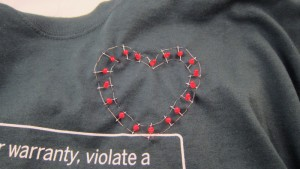How does this compare to conductive thread?

• Pros
• No needle required, because the wire is stiff enough to go through fabric by itself.
• Solderable.
• Available in many stores and relatively cheap.
• Cons
• May break if stretched too much, again because the wire is stiff.
• Can form small curls during sewing if not careful.

Video demo:

So how does this method work? Just follow my illustrated examples below:First, get some 30AWG wire wrap wires,. These can be found in many stores (Sparkfun, Digikey, RadioShack etc). Use a wire stripper to strip the plastic exterior. You can also get 28AWG stringing wires (used for beading) available from craft stores. These wires are un-insulated, so you don’t even need to strip them.Next, pick the fabric or textile you want to sew LEDs onto. I happen to have this awesome Make T-shirt. So let me use that!Sketch your design with a chalk. I am going to have a string of LEDs connected in parallel along a heart shape. Here is my sketch. Not too bad.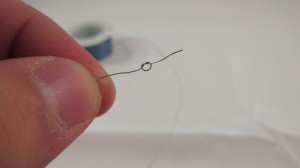Cut a piece of stripped wire, about 20 inch long. At one end, make a small knot and tighten it.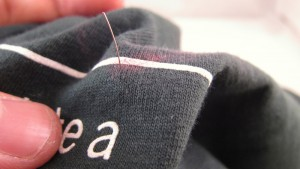Hold the other end using your thumb and index finger, and push it through the fabric, just like how you would use a needle. Since the wire is quite stiff, it can penetrate fabric quite easily.Follow your sketched pattern and move the wire forward, just like how you sew normally. When pulling the wire out, be careful not to form small curls. Use your finger to keep the wire as smooth as possible. Watch the video above for demonstration.This is the outer layer of the heart shape.On the inner side of the fabric, use an electric tape to fix the wire, and also to avoid shorting it with other wires.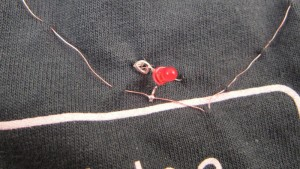One advantage of using the wire is that you can solder electronic components directly onto it. But if you want to avoid soldering, you can still use the wire to sew the component leads to the fabric (in the same way as conductive thread). In this image, the upper lead of the LED is sewed using the wire (without soldering), and the lower lead is soldered.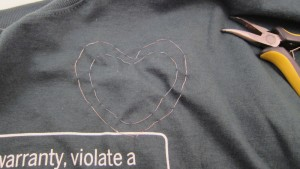Now the inner layer is also finished. I didn’t draw a sketch for the inner layer, so it’s not perfectly parallel to the outer layer 🙁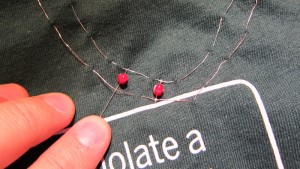Now I will solder LEDs onto the two wires. All LEDs are connected in parallel. The outer wire corresponds to positive lead, and inner wire negative.A good way to keep everything organized is to first prepare all LEDs: bend their leads, and cut the negative leads short. This way, you won’t forget which lead is positive!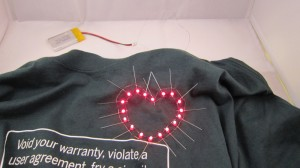Try to keep an even spacing between LEDs, and fix them with a small amount of solder. After this is completed, connect the two wires to a battery to test if all LEDs are working.Now use a wire cutter to cut all excessive leads. I actually like the spikes in the previous image. Looks more artistic :).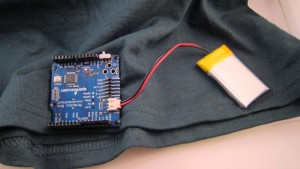To provide programmable LED patterns, I use an Arduino Pro from Sparkfun. I sewed it onto the bottom of the shirt using normal threads.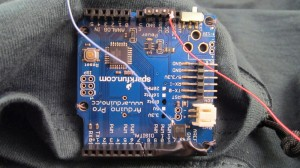I use Arduino Pin 11 to provide PWM control. Since there are 18 LEDs connected in parallel, driving them together requires a current that exceeds the limit of a single IO pin. So I use a BS170 MOSFET to switch the LEDs directly from power supply.Here I am, wearing the Make T-Shirt customized with an LED heart! The LEDs are programmed with three modes: constant on, flashing, and fading. See the video above for demo. Enjoy!

# Learning Electronics — 1. Astable Multivibrator

This is the first article in a series that I call ‘Learning Electronics’. The goal is to learn and experiment with basic electronic circuits. The first circuit I will discuss here is the astable multivibrator. This is a very simple oscillator circuit that can be used to generate square waves. Here is the schematic of a standard astable multivibrator: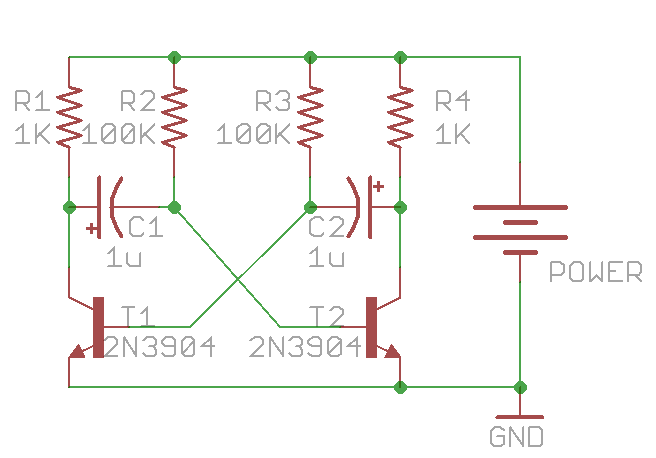It consists of only two NPN transistors (T1 and T2), two capacitors (C1 and C2), and four resistors (R1-R4), organized in a symmetric fashion. The collectors of T1 and T2 are outputs, which provide complement square wave signals. The values of the capacitors and resistors can vary depend on the desired frequency, and their values do not have to be symmetric.

Update: as some readers pointed out, for square wave output, the resistor values should satisfy: R2 / R1 < h_fe, where h_fe is the transistor's current gain (same for R3 and R4). As h_fe is usually around 80 to 250 for 2N3904, the specific values above (R1=1K, R2=100K) are close to the margin. To improve this, consider increasing R1 or decreasing R2. Alternatively, you can replace 2N3904 with BC547, or even a Darlington transistor (e.g. MPSA14), which have higher h_fe values.

##### Principles

So how does the circuit work? To begin, when power is applied, theoretically both T1 and T2 should turn on, since their base pins are connected through resistors (R2 and R3) to Vcc. However, due to small differences in the electric properties, one of the them will turn on slightly earlier than the other. Without loss of generality, let’s assume T2 turns on first. Therefore, T2’s collector begins to conduct and can be thought of as ‘shorted’ to ground. Note that C2’s right lead is connected to T2’s collector, and since C2 is not charged yet, its left lead also has a voltage close to ground. This immediately shuts off T1, i.e. T1 becomes an open circuit. Therefore C1’s left lead is in floating status, and its right lead is connected to T2’s base, which is about 0.7V due to the forward voltage drop of transistors. This forward voltage drop is the same as a diode. So at this moment, the circuit is equivalent to the following:Stage 1

During this period, T2’s collector (output O2) remains low, and C2 begins to charge through R3. Thus the voltage on C2’s left lead will rise, and the rising time depends on R3 x C2. At the same time, C1 also charges, through R1, which is typically a small resistor (e.g. 100-1000 ohm). So C1’s left lead (output O1) will quickly rise up to Vcc and remains high.

As C2 continues to charge, a critical moment will happen when its left lead rise up to 0.7V, at which moment transistor T1 will turn on, and its collector will conduct to ground. Note that since C1’s left lead is connected to T1’s collector, it will also drop to ground voltage. As C1 is fully charged, it’s right lead will suddenly drop to a negative voltage (-Vcc). This will shuts off T2 firmly. Therefore the circuit will suddenly transition to the following equivalent:Stage 2

During this period, output O1 will remain low, and output O2 will quickly rise to Vcc (due to the charging of C2 through a small resistor R4). At the same time, C1 charges through R2 and the voltage on its right lead will rise over time determined by R2 x C1. Now you can predict what’s going to happen: as C1 continues to charge, the next critical moment happens when C1’s right lead rises above 0.7V. At that point, T2 will conduct again while T1 shuts off. The same cycle will repeat. There you go, oscillators!

##### Analysis

Now we have to do some calculus to find out the frequency of the oscillator. Let me focus on output O2. As it alternates between low and high, it approximates a square wave. How to find out the frequency? Note at the end of stage 1, C1 has a voltage of (Vcc – Vb) across it (where Vb = 0.7V). Again, the voltage establishes very quickly because R1 is small. The moment the circuit transitions from stage 1 to stage 2, C1’s right lead has an initial negative voltage: -(Vcc – Vb), and the circuit remains in this stage until that lead rises up to +Vb. So let’s calculate how long this will take. There are ready-made equations to compute this, but here let me derive it from basic differential equations.To start, the problem can be simply stated as follows: a resistor R2 and capacitor C1 are connected in series between Vcc and ground. C1 has an initial voltage of -(Vcc – Vb). How long does it take for C1’s voltage to rise up to +Vb? Let’s assume C1’s voltage is V(t): a function that changes over time. According to the law of capacitor, the change of V(t) is equal to the current flowing through it divided by the capacitance:

How much is the current i(t)? That’s equal to the current flowing through the resistor R2, thus

putting them together:

This is a simple differential equation. We can rearrange it to the following form:

By using the fact that

and integrate both sides, we get:

where K is a constant. Using the knowledge that when t=0, V(t)= -(Vcc – Vb), we find out

so:

Recall that our goal is to find hen V(t) is equal to Vb, so substitute Vb to V(t), and solve for t:

Under the assumption that Vcc is much larger than Vb, this is approximately equal to:

This is how long stage 2 will last. Similarly, stage 1 will last for approximately

Therefore, a full cycle will take

before the next cycle begins. The frequency of the wave is thus:

Viola! We are done!

With the given values in the first schematic (C1=C2=1uF, R2=R3=100K), the frequency is roughly 6.5Hz. These symmetric values can produce a square wave of 50% duty cycle. But they do not have to be symmetric. By using different R2 and R3 (or different C1 and C2), you can easily create waves with any desired duty cycle.

##### Practice

It’s very easy to prototype the circuit on a breadboard. Here is an example: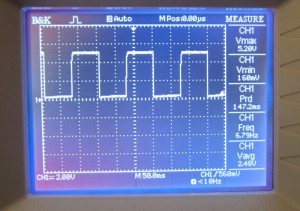Connecting an oscilloscope to output O2, the result shows a decent square wave with 50% duty cycle at about 6.7Hz. Looks quite nice.I also checked the signal on T2’s base pin. The result is on the left. As you can see, the beginning of stage 2 is signaled by the jump to a large negative value. During this stage, C1 charges through R2, so T2’s base voltage rises continuously. As soon as it crosses Vb, T2 conducts, and its base remains at Vb until the start of the next cycle.

Next, let’s blink some LEDs with the oscillator. You can either connect LEDs in series with R1 and R4, or directly to output O1 and O2. Check the video below (the circuit below uses C1=C2=10uF):

Finally, if you want, you can make a printed circuit board, so you can carry it around, or use it to decorate a toy with flashing LEDs. Enjoy!

##### Appendix

Frequency change when connecting LEDs with R1 and R4 in series
As mentioned above, one way to connect LEDs into the multivibrator is by putting them in series with R1 and R4. One interesting thing I noticed is that when doing so the frequency of the LED blinking is much faster than the calculated frequency. So what’s happening? A careful analysis reveals that this is not surprising. The main reason is that because the LED will drop about Vled=2V, C1 and C2 will not be charged all the way to Vcc-Vb anymore. Instead, they are charged to about Vcc-Vb-Vled. Consequently, it takes less time for C1 or C2 to charge from a negative voltage to +Vb, hence the oscillation frequency is faster. The precise formula is actually:

Since Vb+Vled is quite significant compared to Vcc, we cannot make the same approximation as above anymore. For example, with the given values in the first schematic (C1=C2=1uF, R2=R3=100K), and assume Vcc=+5V, the frequency is now roughly 10Hz (compared to 6.5Hz when LEDs are not connected). So this explained why the LED blinking speed seems faster than calculated.